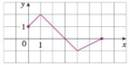Chapter 1.3, Problem 5E### Single Variable Calculus: Early Tr...

8th Edition
James Stewart
ISBN: 9781305270343

#### Solutions

Chapter
Section### Single Variable Calculus: Early Tr...

8th Edition
James Stewart
ISBN: 9781305270343
Textbook Problem

# The graph of f is given. Use it to graph the following functions.(a) y = f ( 2 x ) (b) y = f ( 1 2 x ) (c) y = f ( − x ) (d) y = − f ( − x )(a)

To determine

To sketch: The graph of the function y=f(2x) by using the given graph.

Explanation

The equation y=f(2x) represents that the graph of the function is horizontally shrunk by a factor of 2. Thus, the graph of y=f

(b)

To determine

To sketch: The graph of the function y=f(12x) by using the given graph.

(c)

To determine

To sketch: The graph of the function y=f(x) by using the given graph.

(d)

To determine

To sketch: The graph of the function y=f(x) by using the given graph.

### Still sussing out bartleby?

Check out a sample textbook solution.

See a sample solution

#### The Solution to Your Study Problems

Bartleby provides explanations to thousands of textbook problems written by our experts, many with advanced degrees!

Get Started

#### limnn2+3n2n2+n+1= a) 0 b) 12 c) 1 d)

Study Guide for Stewart's Single Variable Calculus: Early Transcendentals, 8th

#### For

Study Guide for Stewart's Multivariable Calculus, 8th

#### Explain why partial counterbalancing is sometimes necessary.

Research Methods for the Behavioral Sciences (MindTap Course List)